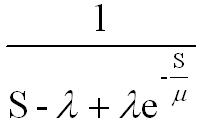# inverse Laplace Transform

Status
Not open for further replies.

##### Full Member level 2
Hello
can anyone tell me the inverse Laplace transform of this relation?well inverse laplace can be done by two ways by using the formula as follows

or if the h(s) given is of the form Nr/Dr
then go for partial fraction , if ROC is not sepceified then u can take the system as causal and find the inverse LT by using basic Laplace transform formule for exp(at) or sin(at) or cos(at) so on

or u can use this if u r confortable

x(t)=(1/(2Πj)*(∫exp(st)X(s)ds)

where the limits of ∫ is σ+j∞ is Upper limit and σ-j∞ is lower limit
usually all the ILTs can b doen by the first method :smile:

---------- Post added at 10:58 ---------- Previous post was at 10:56 ----------

hey perado what are lambda and mu ? r they some constsnts?

•andre_luis

Points: 2